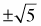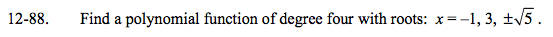### Home > A2C > Chapter 12 > Lesson 12.2.1 > Problem12-88

12-88.

Find a polynomial function of degree four with roots: x = −1, 3,Homework Help ✎Use the roots and the Zero Product Property to write an equation in factored form, then simplify.

P(x) = (x + 1)(x − 3)(x2 − 5) = ?